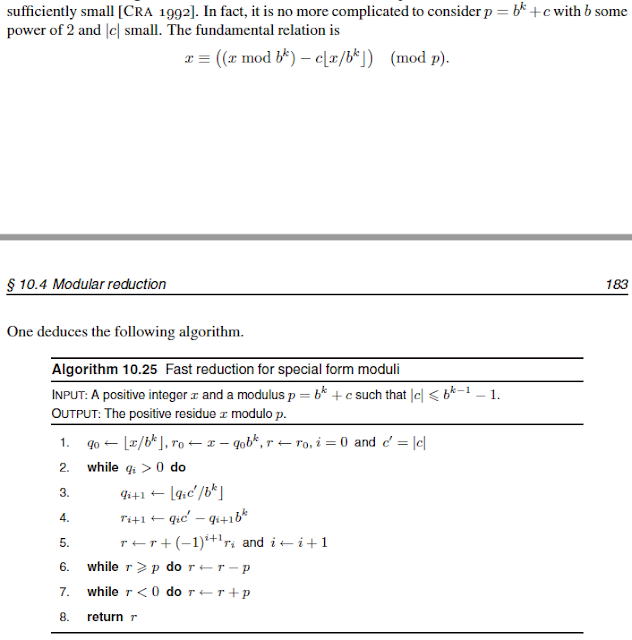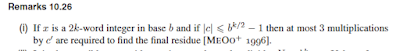How to try to predict the output of Micali-Schnorr Generator (MS-DRBG) knowing the factorization

The article was modified since its publication. Last update was 09/10/2017

See  also Part II and Part III of this series

tl;dr in this post we are going to describe how to try predict the output of Micali-Schnorr Generator (MS-DRBG)  knowing the factorization of the n value. If this sounds like, "why the hell should I care?", you might want to give a look at this great post from Matthew Green about the backdoor in Dual_EC_DRBG. But In a nutshell, quoting Matthew Green :
What I am NOT claiming in this post though is that there is a backdoor in one of this standard.

Introduction

From there I could "track" the challenge that he launched to enthusiastic mathematician.

Mind that I am yes enthusiastic but not a mathematician, but I still decided to accept the challenge :p
And yeah it looks like it took be about two weeks to (partially) solve the riddle.

Micali-Schnorr Generator (MS-DRBG)

So now I "accepted" this challenge, but Hey I do not even know that this Micali-Schnorr Generator (MS-DRBG) existed, so time to study. Googling a bit I found out that other people tried this previously but did not succeed ( I guess), not a good sign, but let's do not this discourage us. The best place where I found the explanation for the Micali-Schnorr Generator was this short pdf (while other books and online resource described it as well).
Trying to keep it simple the MS-DRBG given a number n and an RSA exponent e will output a sequence of bits. The slides from NIST explains this in a really simple way:

The logic behind this is extremely trivial, you start from a secret seed s (this is the internal state of the DRBG) and you will use the n and the e from the standard to do a classic RSA encryption aka (s)^e mod (n) then you will:
• output the less significant bit of (s)^e mod (n)
• and you keep  the most significant bit as the new state for the subsequent iteration
Well that is basically it. Now that we know better MS-DRBG I hope the question from Matthew results simpler, but basically he was asking: will the knowledge of the factorization of n give an advantage such being able to recover the internal state of the DRBG ? To be fair answering no to this question  would be extremely counter intuitive. At the end of the day RSA is completely bound to the secretiveness of the factorization of n and this DRBG is totally RSA, this was what really puzzled me. It must be something but what?
One little thing to note is that it seems that the original definition from Micali and Schnorr and the NIST's  standard differ from the number of bit they output.
The NIST standard is much more conservative and outputs about 1/3 of the RSA computation. While it seems that the original version outputs up to 3/4 of the RSA computation and keeps only 1/4 for the internal state (well this give still 128 bits to recover, still pretty many...).
Well well the second version seems easier, so let's start simple.

Predict the output

Ok now I know the problem statement but I still need to solve it. Let's see a bit of literature. For a reason that hopefully I clarify in another post, thanks to Brian Smith,  I came to know the Handbook of Elliptic and Hyperelliptic Curve Cryptography book. This book isn't only about elliptic curves (the reason why I bought this book BTW) but it also contains explanation of basic algorithms. My initial though was really simple. If I know the factorization of n say I know n = p * qI can maybe somehow use the most lethal weapon any cryptographer can use: the Chinese Remainder Theorem (CRT). I successfully used myself a couple of times already  and . So what I thought is that in any moment I will have the less significant bit of (s)^e mod (n) and the factorization of n = p* q. The idea is really simple: if I can somehow obtain (s)^e mod(p) and (s)^e mod (q) from this partial knowledge I can finally obtain the full  (s)^e mod (n) using CRT!!!!!
So I opened the Handbook of Elliptic and Hyperelliptic Curve Cryptography book at the modular reduction part and I was "attracted" by the Special moduli section. I almost immediately discarded Mersenne primesfrom Handbook of Elliptic and Hyperelliptic Curve Cryptography
because the lack of the 2^128,2^520 interval (the one interesting for cryptography). But turning page I got an "illumination", it talked indeed about Crandall primes of the form p = b^k +c and described an algorithm for reduction for special form modulifrom Handbook of Elliptic and Hyperelliptic Curve Cryptography

Well bingo, looks like is really what I need. Now assuming p and q are both Crandall primes and p = 2^ 256 + c1 amd q = 2^ 256 + c2.

Lets split a bit the algorithm and comment on it:

• q0 = is half unknown (128 bits) and half known (1/3 of the DRBG output, the most significant bits)
• r0 = is the 2/3 of the DRBG output, the less significant bits.
Now is also important to know this:from Handbook of Elliptic and Hyperelliptic Curve Cryptography

Predict the output (updated)

So as said above I spotted an issue on my program that invalidates so far my results. But hey not all of them :p (I hope).
Basically I still claim some less exiting result. Indeed using the technique based on Crandall's primes above I clam that I can predict ~ the 128 less significant bits of (s)^e mod(p) and (s)^e mod (q). If you still do not believe me here is the gist with a POC you can run*:

Now while this is not revolutionary this result is new to me. In order to have a rough idea of why this actually works (most of the time) let see how binary multiplication actually works. The article from Wikipedia contains a good example:

As you see the less significant bits of the result depends only on the less significant bits of the second term.

Now decoding the Crandall's algorithm notation:
• qi+1 = c1 * q0 and taking the 2^256 most significant bits (this is usually a really small number if c1 is small).
• ri+1 = c1 * q0 and taking the 2^256 less significant bit. This can also be written as  c1 * q0 (mod 2^256). Hold on but we know the 128 less significant bits of the second term, and as shown above this means we can also predict the 128 less significant bits of this result.

Now the question is: would knowing the ~ the 128 less significant bits of (s)^e mod(p) and (s)^e mod (q) help to recover the internal state of the DRBG?

Acknowledgement

I would like to thank Matthew Green to give me such a good puzzle :) and Brian Smith for letting me know about  the Handbook of Elliptic and Hyperelliptic Curve Cryptography book.

* I am aware the p and q are not suited for RSA but this is just an example.

OpenSSL Key Recovery Attack on DH small subgroups (CVE-2016-0701)

Usual Mandatory Disclaimer: IANAC (I am not a cryptographer) so I might likely end up writing a bunch of mistakes in this blog post... tl;dr The OpenSSL 1.0.2 releases suffer from a Key Recovery Attack on DH small subgroups . This issue got assigned CVE-2016-0701 with a severity of High and OpenSSL 1.0.2 users should upgrade to 1.0.2f. If an application is using DH configured with parameters based on primes that are not "safe" or not Lim-Lee (as the one in RFC 5114 ) and either Static DH ciphersuites are used or DHE ciphersuites with the default OpenSSL configuration (in particular SSL_OP_SINGLE_DH_USE is not set) then is vulnerable to this attack.  It is believed that many popular applications (e.g. Apache mod_ssl) do set the  SSL_OP_SINGLE_DH_USE option and would therefore not be at risk (for DHE ciphersuites), they still might be for Static DH ciphersuites. Introduction So if you are still here it means you wanna know more. And here is the thing. In my last bl

The Curious Case of WebCrypto Diffie-Hellman on Firefox - Small Subgroups Key Recovery Attack on DH

tl;dr Mozilla Firefox prior to version 72 suffers from Small Subgroups Key Recovery Attack on DH in the WebCrypto 's API. The Firefox's team fixed the issue r emoving completely support for DH over finite fields (that is not in the WebCrypto standard). If you find this interesting read further below. Premise In this blog post I assume you are already knowledgeable about Diffie-Hellman over finite fields and related attacks. If not I recommend to read any cryptography book that covers public key cryptography. Here is a really cool simple explanation by David Wong : I found a cooler way to explain Diffie-Hellman :D pic.twitter.com/DlPvGwZbto — David Wong (@cryptodavidw) January 4, 2020 If you want more details about Small Subgroups Key Recovery Attack on DH I covered some background in one of my previous post ( OpenSSL Key Recovery Attack on DH small subgroups (CVE-2016-0701) ). There is also an academic pape r where we examine the issue with some more rigors.

All your Paypal OAuth tokens belong to me - localhost for the win

tl;dr   I was able to hijack the OAuth tokens of EVERY Paypal OAuth application with a really simple trick. Introduction If you have been following this blog you might have got tired of how many times  I have stressed out the importance of the redirect_uri parameter in the OAuth flow. This simple parameter might be source of many headaches for any maintainer of OAuth installations being it a client or a server. Accepting the risk of repeating myself here is two simple suggestions that may help you stay away from troubles (you can always skip this part and going directly to the Paypal Vulnerability section): If you are building an OAuth client,   Thou shall register a redirect_uri as much as specific as you can i.e. if your OAuth client callback is https://yourouauthclient.com/oauth/oauthprovider/callback then DO register https://yourouauthclient.com/oauth/oauthprovider/callback   NOT JUST h ttps://yourouauthclient.com/ or https://yourouauthclient.com/oauth If